• 最小二乘法 求解
2022-04-04 13:58:56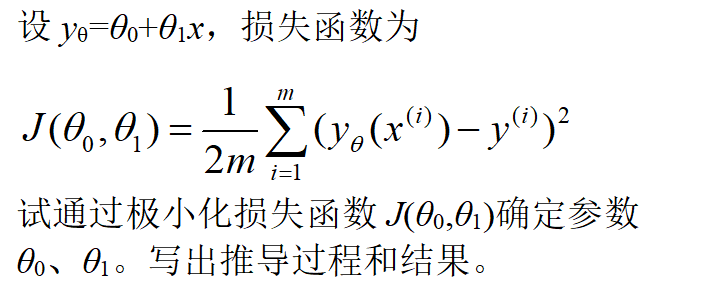参考：《机器学习》西瓜书————周志华

以下为个人笔记，不免有很多细节不对之处。仅供参考！

# 1、原理：

均方误差MES有非常好的几何意义,它对应了常用的欧几里得距离或简称“欧氏距离”(Euclidean distance).基于均方误差最小化来进行模型求解的方法称为“最小二乘法”(least square method).在线性回归中，最小二乘法就是试图找到一条直线,使所有样本到直线上的欧氏距离之和最小.

# 2、模型分析：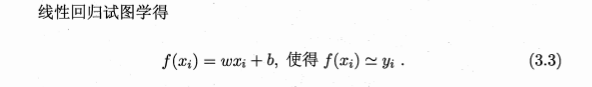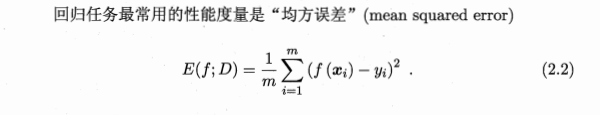如何确定w和b呢?显然,关键在于如何衡量f(z)与y之间的差别，均方误差(2.2)是回归任务中最常用的性能度量,因此我们可试图让均方误差最小化,即：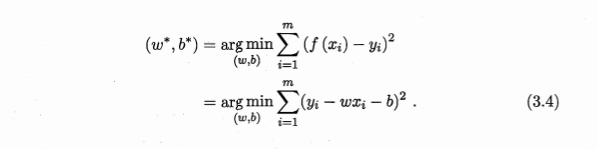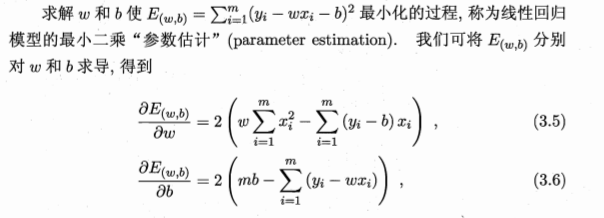然后令式（3.5）（3.6）为零可得到w和b最优解得闭式解：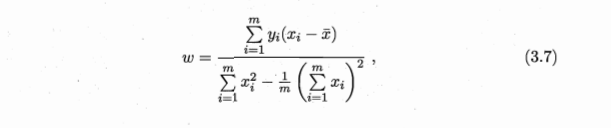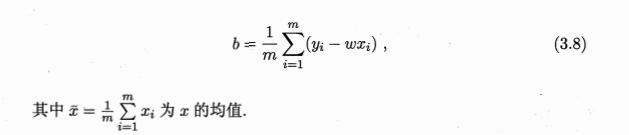# 3、利用最小二乘法求解线性回归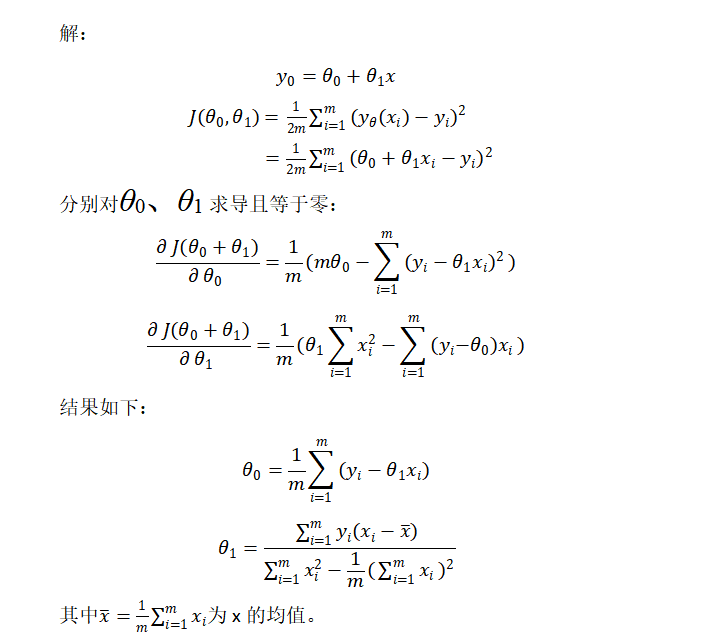关于求偏导过程：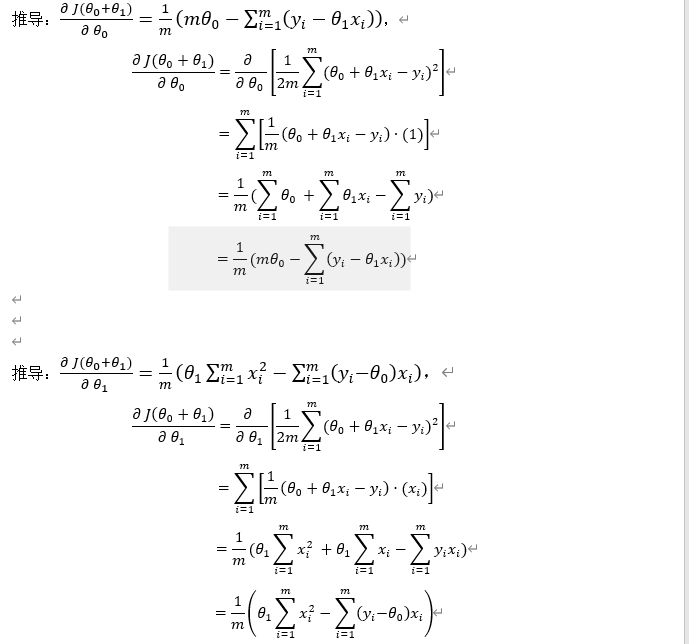推导手稿：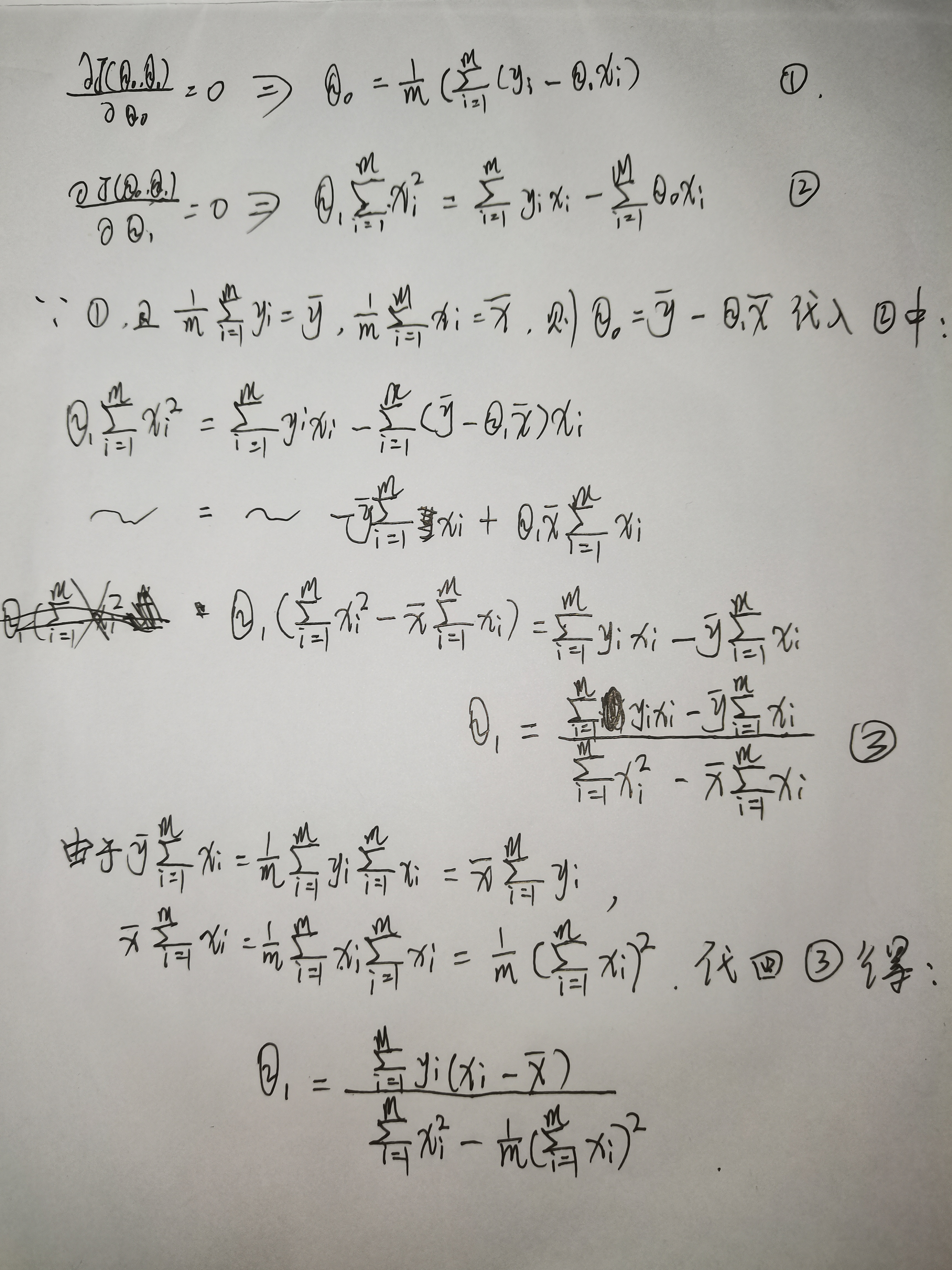注意：

1.前面乘以二分之一是对损失函数求导时消除误差评分项的影响

2.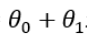,m是一个常数

# 以上是参考周志华西瓜书

更多相关内容
• 采用递推最小二乘法求解超定线性方程组 Ax=b，其中 A 为 mxn 维的已知矩阵，b 为 m 维的已知向量，x 为 n 维的未知向量，其中 n=10，m=10000。A 与 b 中的元素服从独立同 分布的正态分布。绘出横坐标为迭代步数时的...
• 提出了基于最小二乘优化系统的估值方法测量求解光器件物理偏振参量。对于输入偏振态可测或非可测的系统，在建立相应的估值解析方程和适当的测量条件基础上，此测量方案均是简单易行的。对以旋转波片为偏振发生器的...
• ## 最小二乘法求解

千次阅读 2019-07-12 12:25:50
线性最小二乘法 ...非线性最小二乘法求解 https://zhuanlan.zhihu.com/p/42383070 很多问题最终归结为一个最小二乘问题，如SLAM算法中的Bundle Adjustment，位姿图优化等等。求解最小二乘的方法有很多，...

# 线性最小二乘法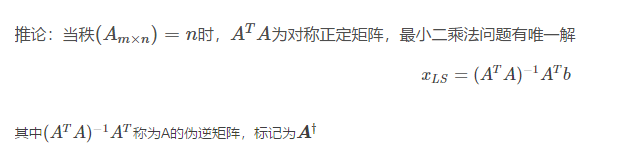可逆矩阵条件：

1.A 为 方阵

2. det(A) 不等于 0  即 A是满秩矩阵 即 A是非奇异矩阵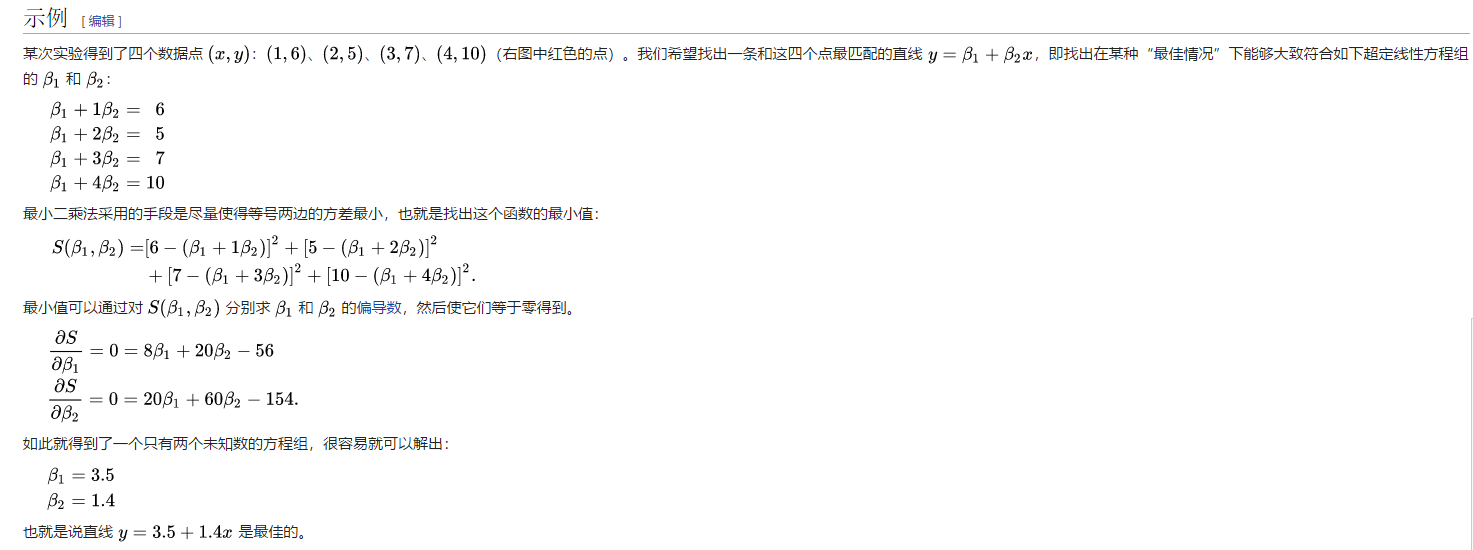# 非线性最小二乘法求解

https://zhuanlan.zhihu.com/p/42383070

## 推导

对于一个非线性最小二乘问题：高斯牛顿的思想是把利用泰勒展开，取一阶线性项近似。带入到(1)式：对上式求导，令导数为0。令，, 式（4）即为求解式（5），便可以获得调整增量。这要求可逆（正定），但实际情况并不一定满足这个条件，因此可能发散，另外步长可能太大，也会导致发散。

使用 H 代替了 二阶导数 海参矩阵，减少了计算量，，，（二阶展开就是海参矩阵）

综上，高斯牛顿法（Gauss-Newton）的步骤为

STEP1. 给定初值STEP2. 对于第k次迭代，计算 雅克比， 矩阵，；根据（5）式计算增量;
STEP3. 如果足够小，就停止迭代，否则，更新.
STEP4. 循环执行STEP2. SPTE3，直到达到最大循环次数，或者满足STEP3的终止条件。

## 编程实现

问题：非线性方程:，给定n组观测数据，求系数.

分析：令,N组数据可以组成一个大的非线性方程组我们可以构建一个最小二乘问题：.

要求解这个问题，根据推导部分可知，需要求解雅克比。

##使用推导部分所述的步骤就可以进行解算。代码实现：

ydsf16/Gauss_Newton_solver​github.com

/**
* This file is part of Gauss-Newton Solver.
*
* Copyright (C) 2018-2020 Dongsheng Yang <ydsf16@buaa.edu.cn> (Beihang University)
*
* Gauss_Newton_solver is free software: you can redistribute it and/or modify
* it under the terms of the GNU General Public License as published by
* the Free Software Foundation, either version 3 of the License, or
* (at your option) any later version.
*
* Gauss_Newton_solver is distributed in the hope that it will be useful,
* but WITHOUT ANY WARRANTY; without even the implied warranty of
* MERCHANTABILITY or FITNESS FOR A PARTICULAR PURPOSE. See the
* GNU General Public License for more details.
*
* You should have received a copy of the GNU General Public License
* along with Gauss_Newton_solver. If not, see <http://www.gnu.org/licenses/>.
*/

#include <iostream>
#include <eigen3/Eigen/Core>
#include <vector>
#include <opencv2/opencv.hpp>
#include <eigen3/Eigen/Cholesky>
#include <eigen3/Eigen/QR>
#include <eigen3/Eigen/SVD>
#include <chrono>

/* 计时类 */
class Runtimer{
public:
inline void start()
{
}

inline void stop()
{
}

inline double duration()
{
return std::chrono::duration_cast<std::chrono::duration<double>>(t_e_ - t_s_).count() * 1000.0;
}

private:
std::chrono::steady_clock::time_point t_s_; //start time ponit
std::chrono::steady_clock::time_point t_e_; //stop time point
};

/*  优化方程 */
class CostFunction{
public:
CostFunction(double* a, double* b, double* c, int max_iter, double min_step, bool is_out):
a_(a), b_(b), c_(c), max_iter_(max_iter), min_step_(min_step), is_out_(is_out)
{}

void addObservation(double x, double y)
{
std::vector<double> ob;
ob.push_back(x);
ob.push_back(y);
obs_.push_back(ob);
}

void calcJ_fx()
{
J_ .resize(obs_.size(), 3);
fx_.resize(obs_.size(), 1);

for ( size_t i = 0; i < obs_.size(); i ++)
{
std::vector<double>& ob = obs_.at(i);
double& x = ob.at(0);
double& y = ob.at(1);
double j1 = -x*x*exp(*a_ * x*x + *b_*x + *c_);
double j2 = -x*exp(*a_ * x*x + *b_*x + *c_);
double j3 = -exp(*a_ * x*x + *b_*x + *c_);
J_(i, 0 ) = j1;
J_(i, 1) = j2;
J_(i, 2) = j3;
fx_(i, 0) = y - exp( *a_ *x*x + *b_*x +*c_);
}
}

void calcH_b()
{
H_ = J_.transpose() * J_;
B_ = -J_.transpose() * fx_;
}

void calcDeltax()
{
deltax_ = H_.ldlt().solve(B_);
}

void updateX()
{
*a_ += deltax_(0);
*b_ += deltax_(1);
*c_ += deltax_(2);
}

double getCost()
{
Eigen::MatrixXd cost= fx_.transpose() * fx_;
return cost(0,0);
}

void solveByGaussNewton()
{
double sumt =0;
bool is_conv = false;
for( size_t i = 0; i < max_iter_; i ++)
{
Runtimer t;
t.start();
calcJ_fx();
calcH_b();
calcDeltax();
double delta = deltax_.transpose() * deltax_;
t.stop();
if( is_out_ )
{
std::cout << "Iter: " << std::left <<std::setw(3) << i << " Result: "<< std::left <<std::setw(10)  << *a_ << " " << std::left <<std::setw(10)  << *b_ << " " << std::left <<std::setw(10) << *c_ <<
" step: " << std::left <<std::setw(14) << delta << " cost: "<< std::left <<std::setw(14)  << getCost() << " time: " << std::left <<std::setw(14) << t.duration()  <<
" total_time: "<< std::left <<std::setw(14) << (sumt += t.duration()) << std::endl;
}
if( delta < min_step_)
{
is_conv = true;
break;
}
updateX();
}

if( is_conv  == true)
std::cout << "\nConverged\n";
else
std::cout << "\nDiverged\n\n";
}

Eigen::MatrixXd fx_;
Eigen::MatrixXd J_; // 雅克比矩阵
Eigen::Matrix3d H_; // H矩阵
Eigen::Vector3d B_;
Eigen::Vector3d deltax_;
std::vector< std::vector<double>  > obs_; // 观测
double* a_, *b_, *c_;

int max_iter_;
double min_step_;
bool is_out_;
};//class CostFunction

int main(int argc, char **argv) {

const double aa = 0.1, bb = 0.5, cc = 2; // 实际方程的参数
double a =0.0, b=0.0, c=0.0; // 初值

/* 构造问题 */
CostFunction cost_func(&a, &b, &c, 50, 1e-10, true);

/* 制造数据 */
const size_t N = 100; //数据个数
cv::RNG rng(cv::getTickCount());
for( size_t i = 0; i < N; i ++)
{
/* 生产带有高斯噪声的数据　*/
double x = rng.uniform(0.0, 1.0) ;
double y = exp(aa*x*x + bb*x + cc) + rng.gaussian(0.05);

/* 添加到观测中　*/
}
/* 用高斯牛顿法求解 */
cost_func.solveByGaussNewton();
return 0;
}
展开全文• 最小二乘法求解一元线性回归 介绍线性回归模型以及简单一元线性回归模型的解法。 通过代码实现最小二乘法求解一元线性回归实例，并对结果进行预测。 一、线性回归 二、回归问题的解决 三、最小二乘法介绍 四、...

### 最小二乘法求解一元线性回归

介绍线性回归模型以及简单一元线性回归模型的解法。

通过代码实现最小二乘法求解一元线性回归实例，并对结果进行预测。

### 一、线性回归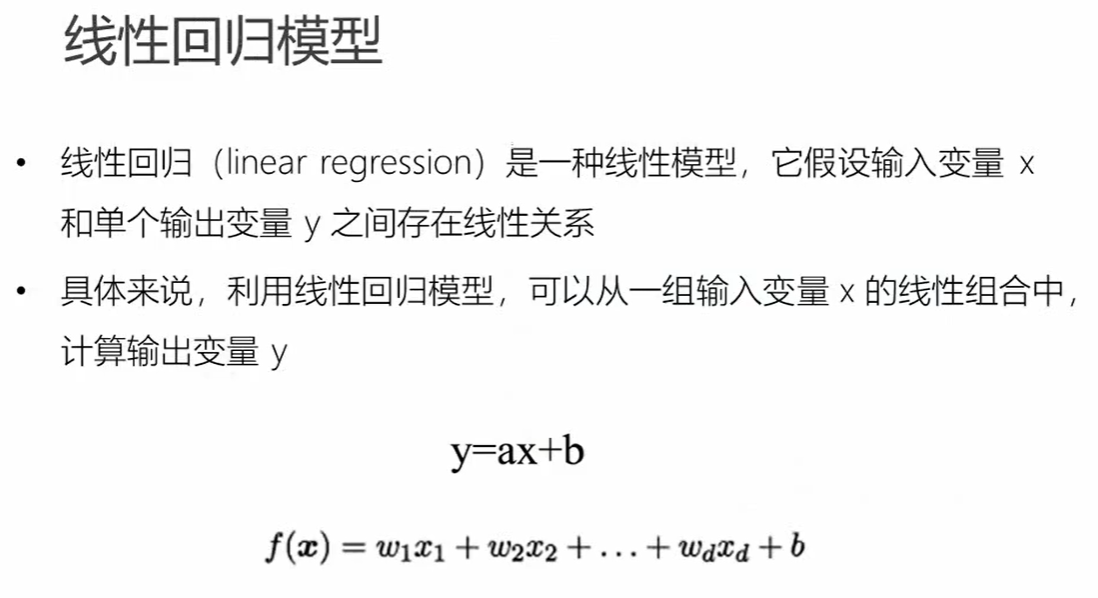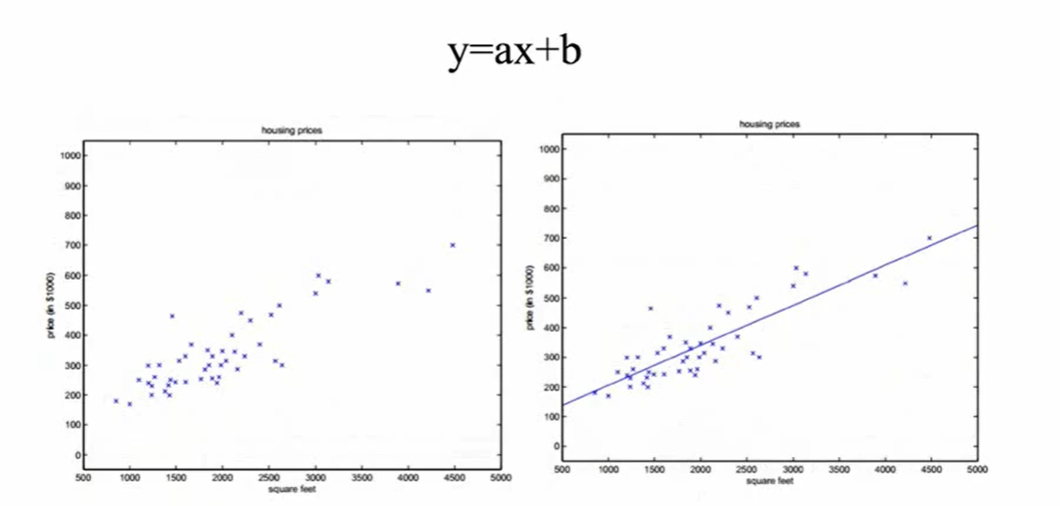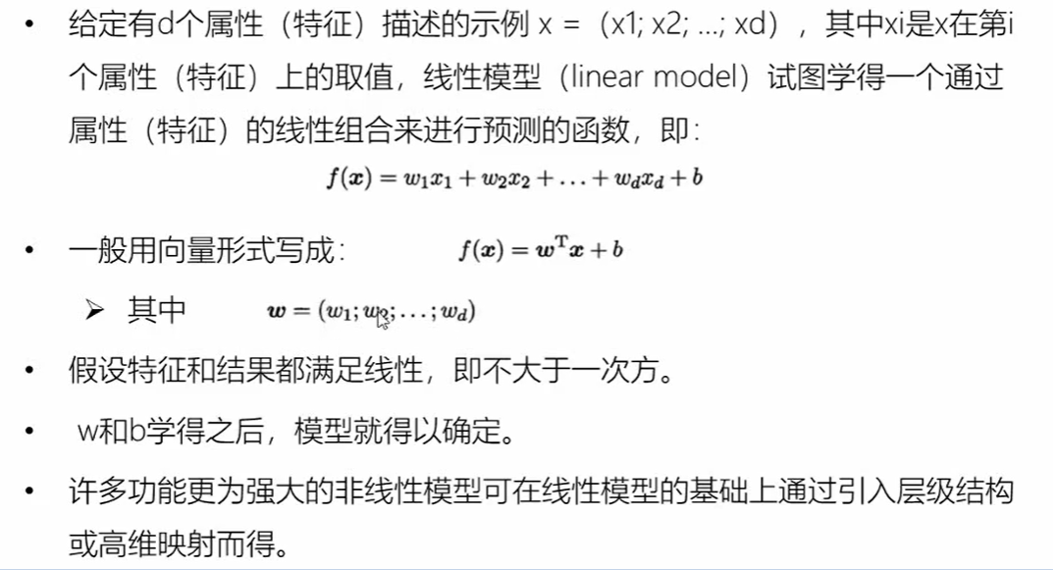### 二、回归问题的解决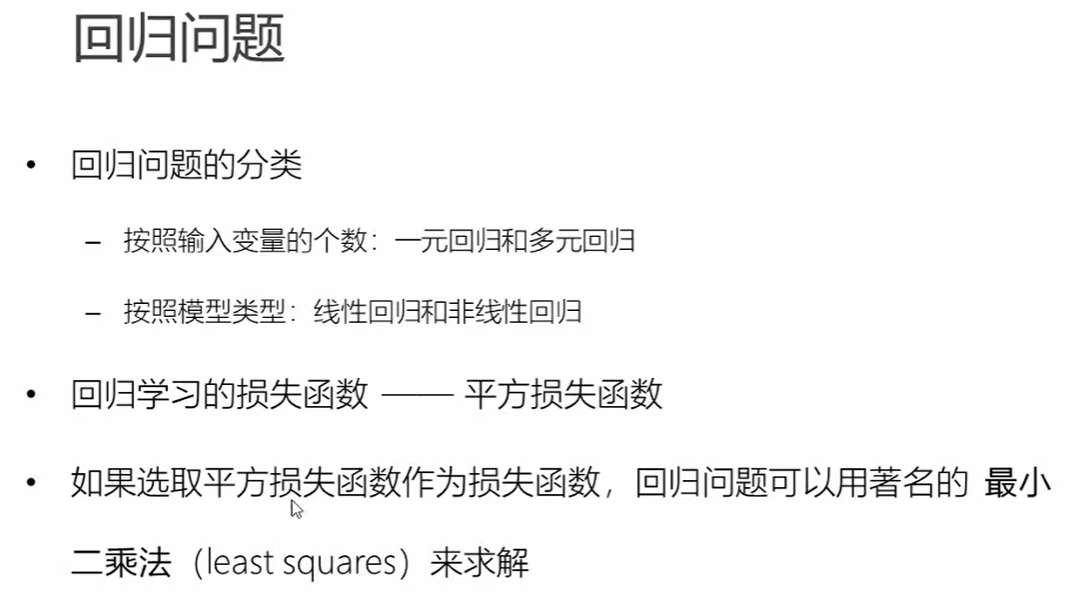### 三、最小二乘法介绍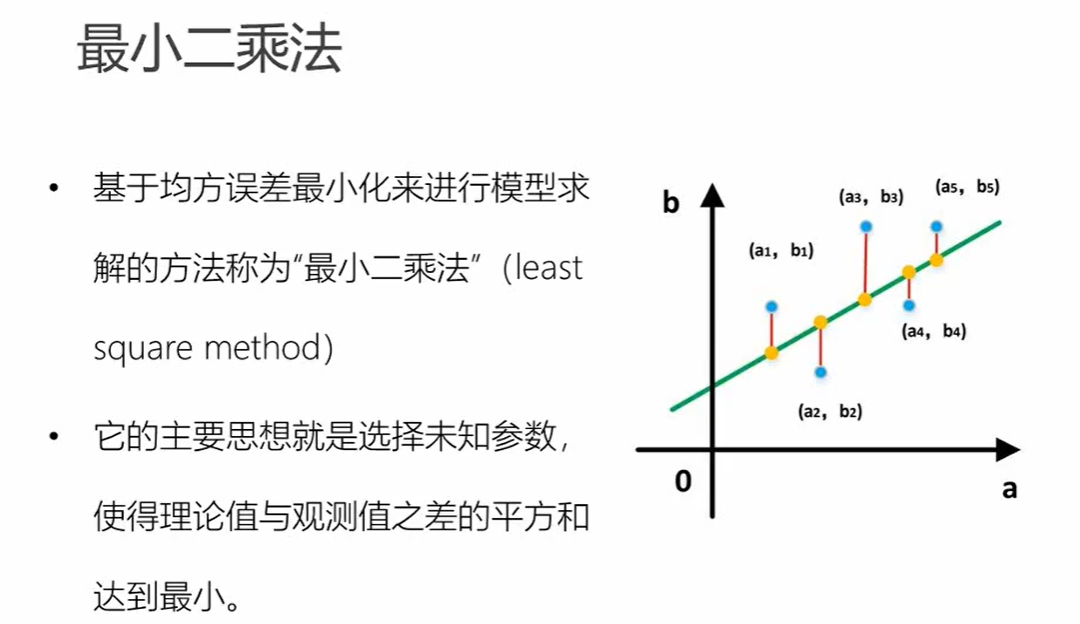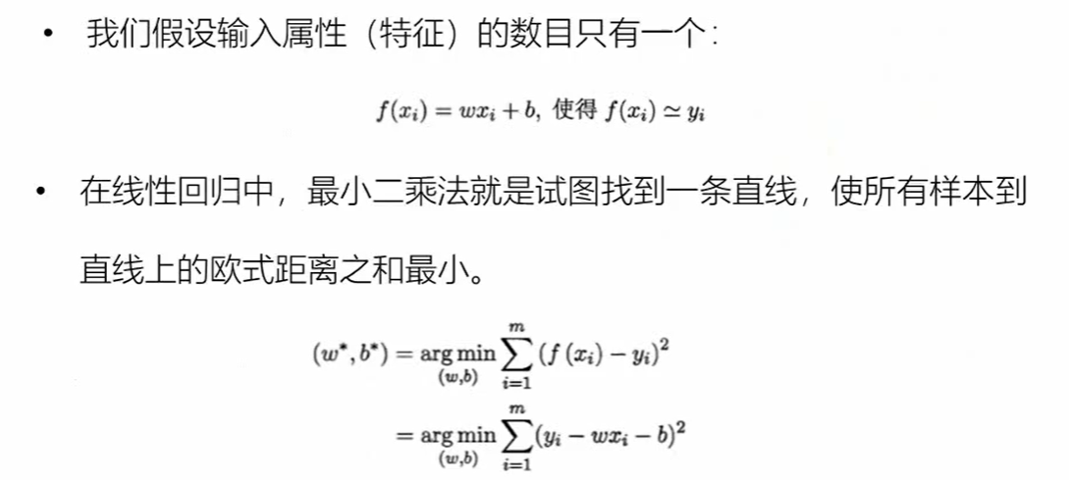### 四、最小二乘法求解线性回归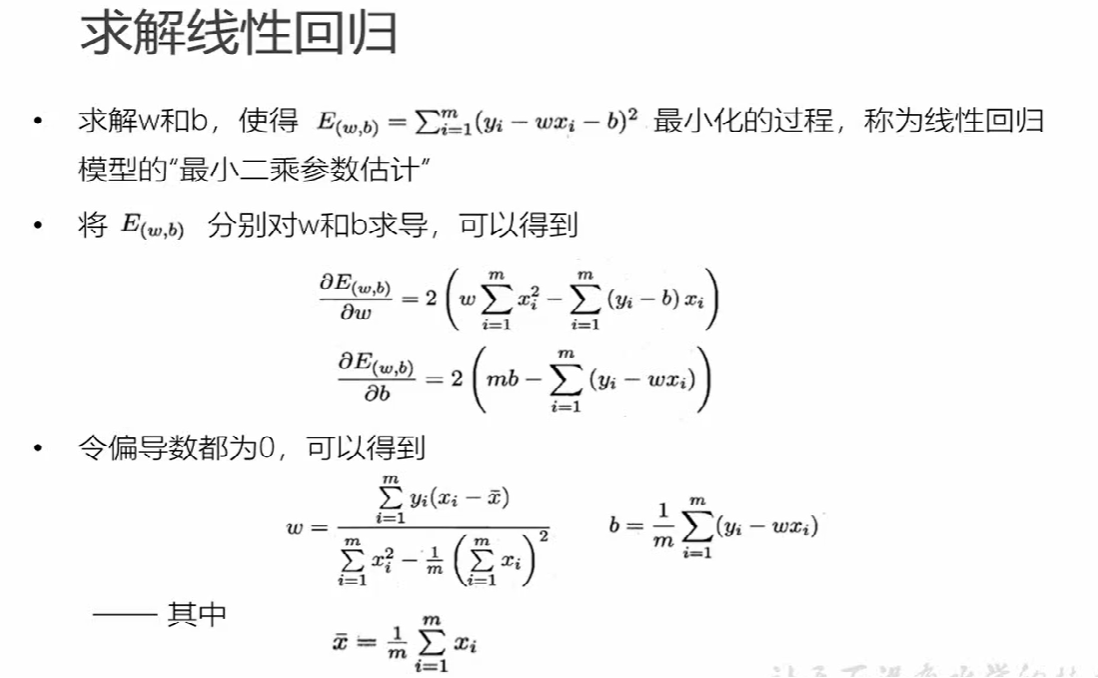### 五、实例验证

##### 案例背景：数据中参数x为学习时间，y为得分。通过最小二乘法求解参数w，b，均方差。并预测x=80时的得分。

数据链接：
链接: https://pan.baidu.com/s/1KVw_9O5o9vqQnpgRNfLGVQ
提取码：8u8e

#### 1.导入数据

# 导入必要库
import numpy as np
import matplotlib.pyplot as plt

points = np.genfromtxt('E:/PythonData/machine_learning/data.csv',delimiter=',')

# 查看前5行数据
points[:5]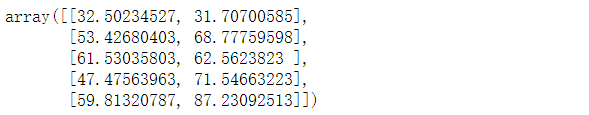#### 2.绘制散点图

# 分别提取points中的x和y数据
x = points[:,0]
y = points[:,1]

# 绘制散点图
plt.scatter(x,y)
plt.show()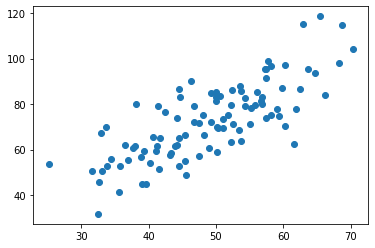#### 3.定义损失函数

# 损失函数是系数w，b的函数，另外还要传入数据x，y
def computer_cost(w,b,points):
total_cost = 0
M = len(points)

# 逐点计算平方损失误差，然后求平均数
for i in range(M):
x = points[i,0]
y = points[i,1]
total_cost +=(y - w *x - b)**2
# 取平均


#### 4.定义算法拟合函数

# 先定义求均值函数
def average(data):
sum = 0
num = len(data)
for i in range(num):
sum+=data[i]
return sum/num

# 定义核心拟合函数
def fit(points):
M = len(points)
x_bar = average(points[:,0])

sum_yx = 0
sum_x2 = 0
sum_delta = 0

for i in range(M):
x = points[i,0]
y = points[i,1]
sum_yx += y*(x-x_bar)
sum_x2 += x**2

# 根据公式计算w
w = sum_yx/(sum_x2 - M*(x_bar**2))

# 再次创建for循环计算b
for i in range(M):
x = points[i,0]
y = points[i,1]
sum_delta += y-w*x
b = sum_delta/M
return w,b


#### 5.测试（得到参数w，b，均方误差）

# 将测试集传入拟合函数中
w,b = fit(points)
print('w is :',w)
print('b is :',b)

cost = computer_cost(w,b,points)
print('cost is ',cost)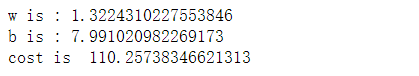#### 6.绘制拟合曲线

plt.scatter(x,y)

# 针对每一个x，绘制出预测的值
pred_y = w*x+b

# 画出拟合曲线，颜色设置为红色
plt.plot(x,pred_y,c='r')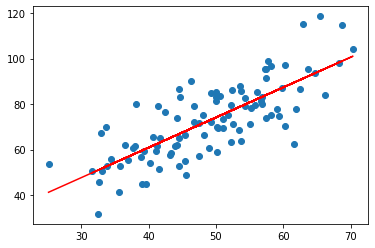#### 7.预测分数

# 给出参数x，得出预测结果
pred_y1 = w*80+b
print(pred_y1)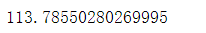展开全文机器学习 线性回归 Python
• 简单的来说，最小二乘法为一类线性算法，将需要求解的系数当作未知数，f(x)与x当作已知数，通过多组对应关系求得系数的方法。所以，最小二乘法仅适合系数为一次项方程式例如：，k与b作为系数是可通过最小二乘法求的...

ci最小二乘法定义（摘抄于百度百科）：基本思路（摘抄于百度百科）：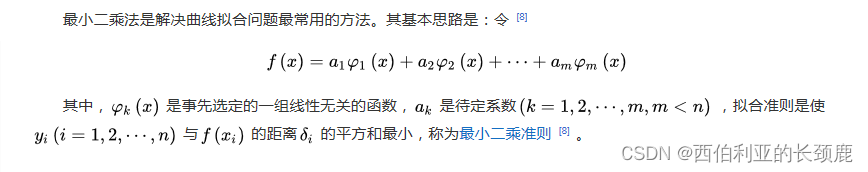简单的来说，最小二乘法为一类线性算法，将需要求解的系数当作未知数，f(x)与x当作已知数，通过多组对应关系求得系数的方法。

所以，最小二乘法仅适合系数为一次项方程式

例如：，k与b作为系数是可通过最小二乘法求的

而：，A作为系数，是不可通过最小二乘法求得的

理论过程：

当圆心为（0,0），半径为r时，可得圆的方程式为：

令圆心为(h,k)时，

圆方程为：

这是在连续数据的情况下，我们将其代入离散数据点的情况：

圆方程为：

展开式为：

为了简化方程式，令

则方程为：

既误差函数为：

对误差函数的二次进行求导，已知E=0，既误差函数的二次方对任何数求导均为0:

所以：

推得：

展开并化简得：

系数放等号左边，常数放等号右边

使用矩阵表示既为

其中

求解矩阵方程既可得到圆心(h,k)

可以利用克莱姆求解矩阵方程

解得：

此方法和推导同样适合多项式方程、椭圆方程等，这边不再做详细介绍

MATLAB代码（为MATLAB R2022版本下.mlapp回调代码）：

            [file,path] = uigetfile;
if isempty(file)
return;
end
if ~contains(file,'.csv')
msgbox("请确定选择文件为<.csv>文件后重试！")
return;
end
filepath=strcat(path,file);
LineAll=table2array(LineData);
LineX=LineAll(:,1);
Liney=LineAll(:,2);

nSum=0;
XSum=0;
YSum=0;
XYSum=0;
X2Sum=0;
Y2Sum=0;

X2YSum=0;
XY2Sum=0;
X3Sum=0;
Y3Sum=0;

N=size(LineX);

for i=1:N
nSum=nSum+1;
XSum=XSum+LineX(i);
YSum=YSum+Liney(i);
XYSum=XYSum+LineX(i)*Liney(i);
X2Sum=X2Sum+LineX(i)*LineX(i);
Y2Sum=Y2Sum+Liney(i)*Liney(i);

X2YSum=X2YSum+LineX(i)*LineX(i)*Liney(i);
XY2Sum=XY2Sum+LineX(i)*Liney(i)*Liney(i);
X3Sum=X3Sum+LineX(i)*LineX(i)*LineX(i);
Y3Sum=Y3Sum+Liney(i)*Liney(i)*Liney(i);
end

C11=2*X2Sum;
C12=2*XYSum;
C13=-1*XSum;
C21=2*XYSum;
C22=2*Y2Sum;
C23=-1*YSum;
C31=2*XSum;
C32=2*YSum;
C33=-1*nSum;

R1=X3Sum+XY2Sum;
R2=X2YSum+Y3Sum;
R3=X2Sum+Y2Sum;

M1=[C11,C12,C13;
C21,C22,C23;
C31,C32,C33;];

M2=[R1;R2;R3];

M3=M1\M2;

A=M3(1);
B=M3(2);
R=sqrt((A^2+B^2-M3(3)));
Resultstring=strcat("圆心(",num2str(A),",",num2str(B),")","半径：",num2str(R));
msgbox(Resultstring);

此文章为个人知识点总结文章， 转载请标注来源。

展开全文机器学习 matlab
• 最小二乘法求解 基于均方误差最小化来进行模型求解的方法称为“最小二乘法”（least square method） 它的主要思想就是选择未知参数，使得理论值与观测值之差的平方和达到最小。 我们假设输入属性（特征）的数目...机器学习 python
• 利用TOA与最小二乘法直接求解，TOA是利用拉格朗日法求解，而且里面的定位图解做得特别好，分别有一点定位，多点定位的的三维视觉图。(TOA is directly solved by least squares method(Innovativeness),TDOA is ...
• 关于视觉SLAM14讲中的最小二乘法求解超定方程1、超定方程组2、最小二乘法求解 1、超定方程组 粗糙的解释：方程的个数比未知数还多。 超定方程组是指方程个数大于未知量个数的方程组。对于方程组Ra=y，R为n×m矩阵，...
• 可用于求非线性方程的最优解，设定初始值的范围，通过优化模型计算出满足范围条件下的最优解。
• 最小二乘法快速解决方程组求解问题 给定方程组系数 旧能快速求出解级
• ## 最小二乘法求解步骤

万次阅读 多人点赞 2019-09-03 23:02:38
目标：线性回归问题，找到最佳参数使得损失函数最小 一、损失函数定义 线性方程：y=ax+by=ax+b y=ax+b 对于每个样本点 x(i)x(i) x^{(i)} ，其预测值为 y^(i)=ax(i)+by^(i)=ax(i)+b \hat y^{(i)}=ax^{(i)}+b ...
• 利用labview制作的简单最小二乘法求解线性方程工具 简单易操作 纯软件制作
• 最小二乘法求解矛盾方程组 参考：最小二乘法求解矛盾方程组 - 知乎 由线性代数理论可知，在求解线性方程组时，当方程式得个数多于未知数的个数时，方程组往往无解，此类方程组称为矛盾方程组（也叫超定方程组） ...
• 最小二乘法求解线性方程组与伪逆 对于线性方程组Ax=bAx=bAx=b的求解。 如果A是可逆的，我们可以通过方程式左右两边乘A−1A^{-1}A−1求解： x=A−1bx=A^{-1}bx=A−1b 但是如果AAA是不可逆的方阵或非方阵呢？ 这时可以...
• 参考代码：运行结果：1.2 3.60.410.8--------董付国老师Python系列教材--------1）《Python程序设计（第2版）》（2018年7月第7次印刷）2）《Pyt...程序设计 编程语言 python
• 一个最小的最小二乘法求解器，针对反复出现的小型密集问题的最小化，可提供极佳的性能。 为什么选择微型求解器？ 以下是Tiny Solver与其他最小二乘最小化实现的不同之处 用户的成本函数直接内联到Levenberg-...
• 1原理 统计学习方法21页 2实现 #n次多项式的...array**(i+1)).reshape((m,1))]) from numpy.linalg import solve #求解系数得到最小的误差 X = solve(np.dot(A.T,A),np.dot(A.T,y_array.T)) print(X) 作图 自己实现看吧
• 使用最小二乘法求解高阶线性方程组，方程组用于位置参数辨识
• 导航与定位问题，泰勒展开法与最小二乘法求解TDOA
• 最小二乘法求解傅里叶级数系数 **自己推导的，如有错误，请大家批评指出 ** 题目 Solution
• 关于递推最小二乘法，网上各种资料，让人莫衷一是，故整理其重要部分，述而不作，说的就是我了！ 最后附MATLAB代码，实测可用 概述 递推最小二乘法的引入（具体见知乎文章） 关键公式 递推公式如下所示（推导见...matlab
• 用于绘制和研究使用最小二乘法求解 ODE 的结果的脚本。 Rnorm 没有被微分以获得系数，而是我们搜索最小化 Rnorm 的 Cj。matlab机器学习 多元线性回归
• 基于jupyter notebook的python编程—–利用梯度下降算法求解多元线性回归方程，并与最小二乘法求解进行精度对比目录一、梯度下降算法的基本原理1、梯度下降算法的基本原理二、题目、表格数据、以及python环境搭建1、...
• 假设 ...m，这就是一个超定方程了，理论上无解，但是我们可以求得最小二乘意义下的解 求解过程 min∣∣AX∣∣22min||AX||^2_2min∣∣AX∣∣22​ ∣∣AX∣∣22=(AX)T(AX)=XTATAX||AX||^2_2=(AX)^T(...最小二乘 超定方程
• Python 最小二乘法求解线性回归模型 机器学习线性回归模型 线性回归（linear regression）是一种线性模型，它假设输入变量 x 和单个输出变量 y 之间存在线性关系 具体来说，利用线性回归模型，可以从一组输入变量......